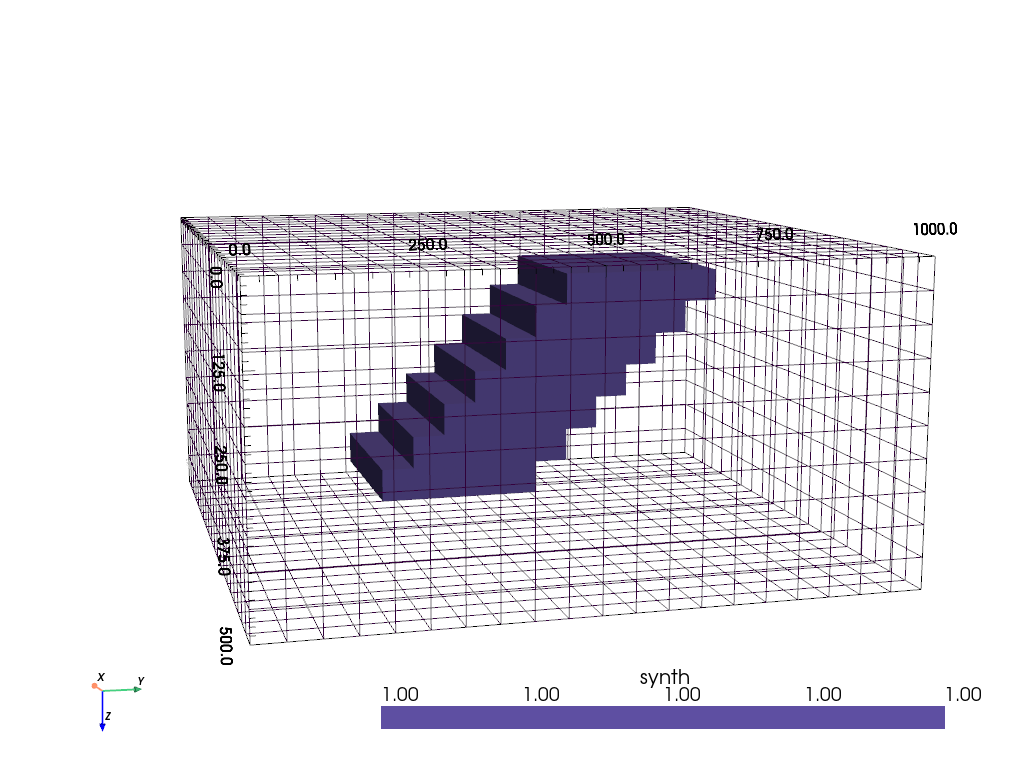# 3D gravity modelling and inversion#

Based on the synthetic model of Li & Oldenburg (1998), we demonstrate 3D inversion of magnetic data. The forward operator bases on the formula given by Holstein et al. (2007).

In the following, we will build the model, create synthetic data, and do inversion using a depth-weighting function as outlined in the paper.

import numpy as np
import matplotlib.pyplot as plt
import pygimli as pg
from pygimli.viewer import pv
from pygimli.physics.gravimetry import GravityModelling


## Synthetic model and data generation#

The grid is 1x1x0.5 km with a spacing of 50 m.

dx = 50
x = np.arange(0., 1001, dx)
y = np.arange(0., 1001, dx)
z = np.arange(0., 501, dx)
grid = pg.createGrid(x, y, z)
print(grid)

Mesh: Nodes: 4851 Cells: 4000 Boundaries: 12800


We create a 3D matrix that is later filled as vector into the grid. The model consists of zeros and patches of 5x6 cells per depth slice that are shifted by one cell for subsequent cells.

v = np.zeros((len(z)-1, len(y)-1, len(x)-1))
for i in range(7):
v[1+i, 11-i:16-i, 7:13] = 1

grid["synth"] = v.ravel()


We show the model making use of the pyvista package that can be called by pygimli.show(). The mesh itself is shown as a wireframe, the anomaly is plotted as a surface plot using a threshold filter. After the first call with hold=True, the plotter is used to draw any subsequent plots that can also be slices or clips. Moreover, the camera position is set so that the vertical axis is going downwards (x is Northing and y is Easting as common in magnetics).

pl, _ = pg.show(grid, style="wireframe", hold=True)
pv.drawMesh(pl, grid, label="synth", style="surface", cMap="Spectral_r",
filter={"threshold": dict(value=0.05, scalars="synth")})
pl.camera_position = "yz"
pl.camera.roll = 90
pl.camera.azimuth = 180 - 15
pl.camera.elevation = 10
pl.camera.zoom(1.2)
_ = pl.show()For the computation of the total field, we define the global magnetic field using the IGRF (total field, inclination and declination) settings given in the paper. Any global field can also be retrieved by the pyIGRF module.

xx, yy = np.meshgrid(x, y)
points = np.column_stack((xx.ravel(), yy.ravel(), -np.ones(np.prod(xx.shape))))
fop = GravityModelling(grid, points)
data = fop.response(grid["synth"])
noise_level = 0.1
data += np.random.randn(len(data)) * noise_level
plt.contourf(yy, xx, np.reshape(data, xx.shape))
plt.colorbar();[++++++++++++++++ 100% ++++++++++++++++] 441 of 441 complete

<matplotlib.colorbar.Colorbar object at 0x7ff3741e5220>


## Depth weighting#

In the paper of Li & Oldenburg (1996), they propose a depth weighting of the constraints with the formula

$w_z = \frac{1}{(z+z_0)^{3/2}}$
# depth weighting
bz = np.array([b.center().z() for b in grid.boundaries() if not b.outside()])
bn = np.array([b.norm().z() for b in grid.boundaries() if not b.outside()])
z0 = 100
wz = z0 / (bz+z0)**1
# wz = z0 / (pg.z(grid.cellCenters())+z0)


## Inversion#

The inversion is rather straightforward using the standard inversion framework pygimli.Inversion.

inv = pg.Inversion(fop=fop, verbose=True)  # , debug=True)
inv.modelTrans = pg.trans.TransCotLU(-2, 2)
# inv.setRegularization(correlationLengths=[500, 500, 100])
inv.setConstraintWeights(wz)
invmodel = inv.run(data, absoluteError=noise_level, lam=3e4,  # zWeight=0.3,
startModel=0.1, verbose=True)
grid["inv"] = invmodel

fop: <pygimli.physics.gravimetry.GravityModelling.GravityModelling object at 0x7ff3d5a89950>
Data transformation: <pygimli.core._pygimli_.RTrans object at 0x7ff3869ca0a0>
Model transformation: <pygimli.core._pygimli_.RTransCotLU object at 0x7ff3741e7ea0>
min/max (data): -462/-14.53
min/max (error): 0.02%/0.69%
min/max (start model): 0.1/0.1
--------------------------------------------------------------------------------
--------------------------------------------------------------------------------
inv.iter 1 ... chi² = 1413.66 (dPhi = 99.83%) lam: 30000.0
--------------------------------------------------------------------------------
inv.iter 2 ... chi² = 22.61 (dPhi = 91.12%) lam: 30000.0
--------------------------------------------------------------------------------
inv.iter 3 ... chi² = 1.1 (dPhi = 16.1%) lam: 30000.0
--------------------------------------------------------------------------------
inv.iter 4 ... chi² = 1.08 (dPhi = 0.08%) lam: 30000.0
################################################################################
#                 Abort criteria reached: dPhi = 0.08 (< 2.0%)                 #
################################################################################


## Visualization#

For showing the model, we again use the threshold filter with a value of 0.02. For comparison with the synthetic model, we plot the latter as a wireframe.

ftr = dict(value=0.5, scalars="synth")
pl, _ = pg.show(grid, label="synth", style="wireframe",
filter={"threshold": ftr}, hold=True, backend="trame")
ftr = dict(value=0.3, scalars="inv")
pv.drawMesh(pl, grid, label="inv", style="surface", filter={"threshold": ftr})
pl.camera_position = "yz"
pl.camera.roll = 90
pl.camera.azimuth = 180
pl.camera.zoom(1.2)
_ = pl.show()The model can outline the top part of the anomalous body and its lateral extent, but not its depth extent due to the ambiguity of gravity. We use a vertical slice to illustrate that.

slice = pg.meshtools.extract2dSlice(grid, origin=[500, 500, 0], normal=[1, 1, 0])
_, ax = plt.subplots(ncols=2, sharex=True, sharey=True)
pg.show(slice, "synth", ax=ax)
pg.show(slice, "inv", ax=ax)
ax.set_ylim(ax.get_ylim()[::-1])(500.0, -1.4210854715202004e-14)